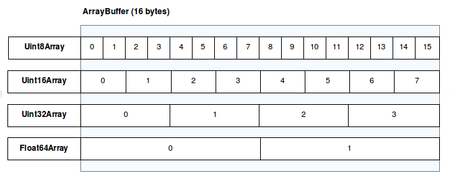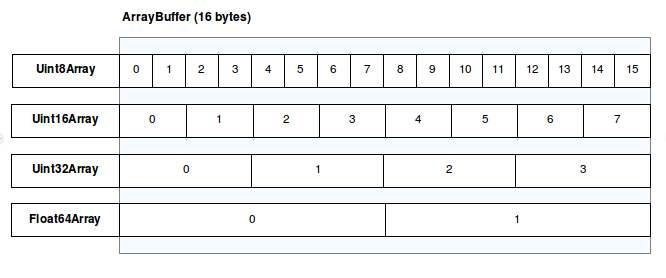Amin## TypedArray 还是 DataView: 理解字节序

### 不喜长文看此处

`ArrayBuffer` 主要用来高效快速的访问二进制数据，比如 WebGL , Canvas 2D 或者 Web Audio 所使用的数据。在这些场景下，你通常想以充分利用硬件性能的方式，或者最容易通过网络传输的方式来存储数据。

### 起底 TypedArrays 和 ArrayBuffer

1. ArrayBuffer，设计用于保存给定数量的二进制数据的数据结构。

2. TypedArray，操作ArrayBuffer的一种视图，每一项都是相同的大小和类型。

3. DataView，操作ArrayBuffer的另外一种视图，不同的地方是每一项可以有自己的大小和类型.

``````var buffer = new ArrayBuffer(2) // array buffer for two bytes
var bytes = new Uint8Array(buffer) // views the buffer as an array of 8 bit integers

bytes = 65 // ASCII for 'A'
bytes = 66 // ASCII for 'B'

``````

``````var blob = new Blob([buffer], {type: 'text/plain'})
var dataUri = window.URL.createObjectURL(blob)
window.open(dataUri)

``````

###哪种方式更好？字节序，第一个部分：

MDN中这张热心肠的表格（叫它红领巾吧）能很好的阐释这个想法：``````var buffer = new ArrayBuffer(2) // array buffer for two bytes
var word = new Uint16Array(buffer) // views the buffer as an array with a single 16 bit integer

var value = (65 << 8) + 66 // we shift the 'A' into the upper 8 bit and add the 'B' as the lower 8 bit.
word = value // write the 16 bit (2 bytes) into the typed array

// Let's create a text file from them:
var blob = new Blob([buffer], {type: 'text/plain'})
var dataUri = window.URL.createObjectURL(blob)
window.open(dataUri)

``````

``````65 decimal = 01 00 00 01 binary
66 decimal = 01 00 00 10 binary

// what we did when we wrote into the Uint8Array:
01 00 00 01 01 00 00 10
<bytes-> <bytes->

// what we did when we created the 16-bit number:
var value = (01 00 00 01 00 00 00 00) + 01 00 00 10
= 01 00 00 01 01 00 00 10

``````

``````var buffer = new ArrayBuffer(2)
// create two typed arrays that provide a view on the same ArrayBuffer
var word = new Uint16Array(buffer) // this one uses 16 bit numbers
var bytes = new Uint8Array(buffer) // this one uses 8 bit numbers

var value = (65 << 8) + 66
word = (65 << 8) + 66
console.log(bytes) // will output [66, 65]
console.log(word === value) // will output true

``````

``````var word = new Uint16Array(buffer)
word = value // 如果 isLittleEndian 没有被显式的设置,  isLittleEndian的值会被设置成true或者false.

``````

### 写入 ArrayBuffers 的另一种方式：DataView

Size in byte Description

2 Identifier “BM” for Bitmap image

4 Size of the image in byte

2 Reserved

2 Reserved

4 Offset (in bytes) between the end of the header and the pixel data

``````var buffer = new ArrayBuffer(14)
var view = new DataView(buffer)

view.setUint8(0, 66)     // Write one byte: 'B'
view.setUint8(1, 67)     // Write one byte: 'M'
view.setUint32(2, 1234)  // Write four byte: 1234 (rest filled with zeroes)
view.setUint16(6, 0)     // Write two bytes: reserved 1
view.setUint16(8, 0)     // Write two bytes: reserved 2
view.setUint32(10, 0)    // Write four bytes: offset

``````

``````Byte  |    0   |    1   |    2   |    3   |    4   |    5   | ... |
Type  |   I8   |   I8   |                I32                | ... |
Data  |    B   |    M   |00000000|00000000|00000100|11010010| ... |

``````

``````var buffer = new ArrayBuffer(2) // array buffer for two bytes
var view = new DataView(buffer)

var value = (65 << 8) + 66 // we shift the 'A' into the upper 8 bit and add the 'B' as the lower 8 bit.
view.setUint16(0, value)

// Let's create a text file from them:
var blob = new Blob([buffer], {type: 'text/plain'})
var dataUri = window.URL.createObjectURL(blob)
window.open(dataUri)

``````

DataView.prototype.setUint16 ( byteOffset, value [ , littleEndian ] )

1. 将 this 赋值给 v.

2. 如果 littleEndian 没有被指定, littleEndian 设置为 false.

3. 返回 SetViewValue(v, byteOffset, littleEndian, “Uint16”, value).

(我太机智了（机智脸）.)

Khronos 团队在现已过时的 原始规范提案 甚至明确的指出:

DataView 的操作基于指定的端序 (大端字节序或者小端字节序).

• TypedArray 使用系统的端序。

• DataView 默认为大端序.

### 结论

`TypedArray` 的目的是提供一种方法来组合二进制数据以便在同一系统上使用 - 因此它选择特定的字节序会更好。

• 假定系统是小端序是安全的。

• TypedArrays 非常适合创建二进制数据，例如传递给 Canvas2D ImageData 或 WebGL。

• DataView 是一种安全的方式来处理接收到的或者发送到其他系统上的二进制数据。